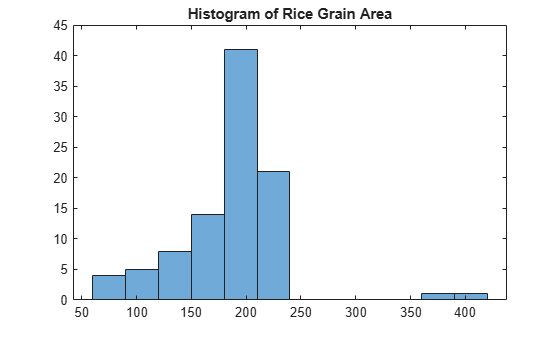# 校正亮度不均匀问题并分析前景对象

### 预处理图像

```I = imread('rice.png'); imshow(I)````se = strel('disk',15)`
```se = strel is a disk shaped structuring element with properties: Neighborhood: [29x29 logical] Dimensionality: 2 ```

```background = imopen(I,se); imshow(background)``````I2 = I - background; imshow(I2)```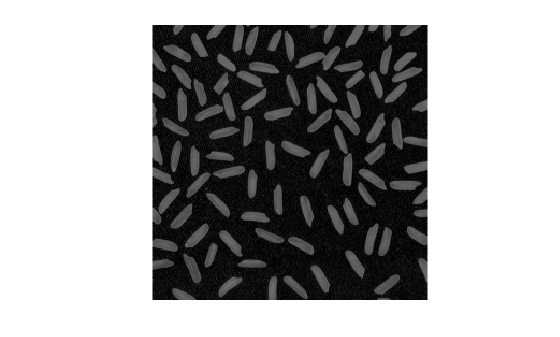```I3 = imadjust(I2); imshow(I3)```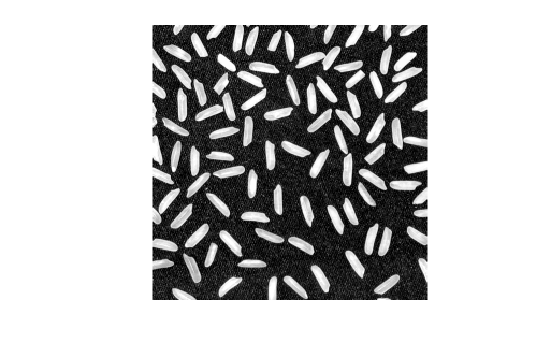`I2 = imtophat(I,strel('disk',15));`

```bw = imbinarize(I3); bw = bwareaopen(bw,50); imshow(bw)```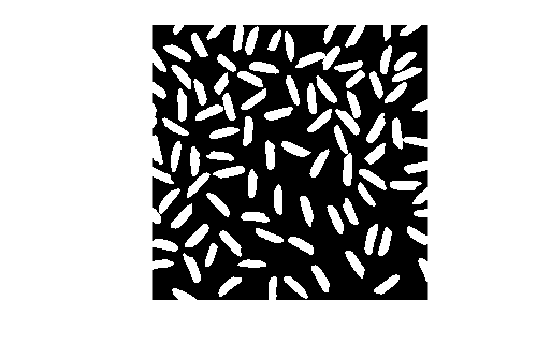### 识别图像中的对象

`cc = bwconncomp(bw,4)`
```cc = struct with fields: Connectivity: 4 ImageSize: [256 256] NumObjects: 95 PixelIdxList: {1x95 cell} ```
`cc.NumObjects`
```ans = 95 ```

```grain = false(size(bw)); grain(cc.PixelIdxList{50}) = true; imshow(grain)```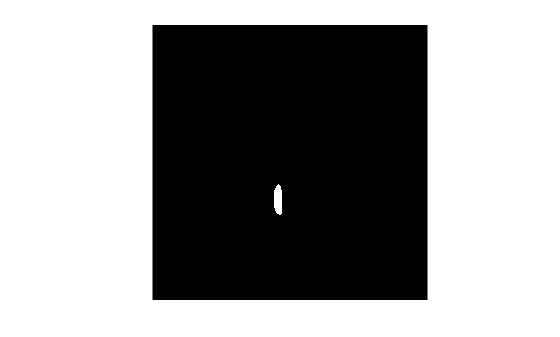```labeled = labelmatrix(cc); whos labeled```
``` Name Size Bytes Class Attributes labeled 256x256 65536 uint8 ```

```RGB_label = label2rgb(labeled,'spring','c','shuffle'); imshow(RGB_label)```### 计算基于面积的统计量

`graindata = regionprops(cc,'basic')`
```graindata=95×1 struct array with fields: Area Centroid BoundingBox ```

`grain_areas = [graindata.Area];`

`grain_areas(50)`
```ans = 194 ```

`[min_area, idx] = min(grain_areas)`
```min_area = 61 ```
```idx = 16 ```
```grain = false(size(bw)); grain(cc.PixelIdxList{idx}) = true; imshow(grain)``````histogram(grain_areas) title('Histogram of Rice Grain Area')```